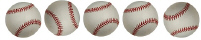KNBT1
 Name:    KNBT1

Multiple Choice
Identify the choice that best completes the statement or answers the question.

1.

Which equation shows this picture?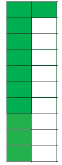a. 12 c. 10 + 1 = 11 b. 11 - 1 = 10 d. 11 = 10 - 1

2.

Which picture shows 10 ones plus 4 more ones?
 a.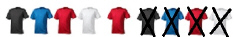c.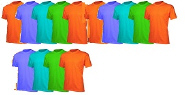b.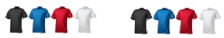d.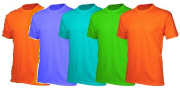3.

Which equation below would be shown by this picture?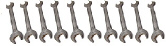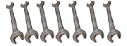a. 17 ones c. 2 tens b. 1 ten and 5 ones d. 1 ten and 7 ones

4.

Which picture shows:  14 - 3 = 11?
 a.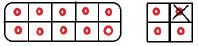c.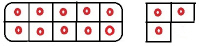b.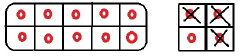d.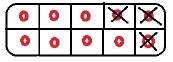5.

Which picture shows 10 ones and 5 more ones?
 a.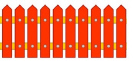c.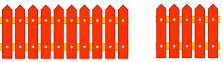b.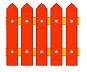d.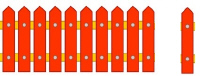6.

Which equation below shows this number:  10
 a. one 10 and 5 more ones c. 10 ones and 10 more ones b. one 10 and 0 ones d. 10 ones minus 1 one

7.

Which equation below shows this number:  19
 a. One 10 and 9 ones c. 20 ones b. 2 tens and 0 ones d. One ten and 5 ones

Multiple Response
Identify one or more choices that best complete the statement or answer the question.

8.

Choose the two pictures that, when put together, would show this equation: 10 + 8 = 18
 a.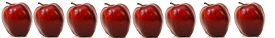c.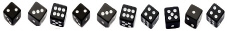b.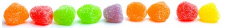d.